# Cross-price elasticity of demand

## Definition

Suppose$A$ and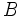$B$ are two commodities. The cross-price elasticity of demand of$A$ with respect to$B$ measures the fractional change in the demand of$A$ in response to a fractional change in the unit price of$B$. Note that the price of$A$ is not changed in the process.

Formally, if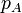$p_A$ and$p_B$ denote the unit prices of$p_A$ and$p_B$ and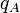$q_A$ and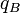$q_B$ denote the quantities demanded for$A$ and$B$, the cross-price elasticity is given by any of the following four equivalent formulations:$\! \frac{p_B dq_A}{q_A dp_B} = \frac{dq_A/q_A}{dp_B/p_B} = \frac{dq_A/dp_B}{q_A/p_B} = \frac{d(\log (q_A))}{d(\log (p_B))}$

Note that although only$q_A$ and$p_B$ appear in the expression for cross-price elasticity, the value$p_A$ could also affect the value of cross-price elasticity. More specifically, the formula makes sense against a backdrop of the value of$p_A$ and all the other determinants of demand.

### Interpretation in terms of demand curve

The cross-price elasticity of demand cannot be computed by looking at any single instance of the usual demand curve or logarithmic demand curve for either$A$ or$B$. Rather, it measures the speed of expansion/contraction of the demand curve for$A$ with respect to a price change in$B$.

The price of$B$ is possibly one of the determinants of demand for the quantity demanded of$A$. The price of$B$ is thus one of the exogenous parameters to the demand curve of$A$. A change in this parameter leads to a shift (expansion or contraction) in the demand curve for$A$ and the magnitude of this shift at the price level$p_A$ is what the cross-price elasticity measures.

## Properties

For the entire discussion, we assume that both$A$ and$B$ are ordinary goods (quantity demanded decreases with increase in price) and normal goods (quantity demanded increases with increase in disposable income). In the absence of these assumptions, more interesting and complicated variations of the possibilities below can occur.

### Factors affecting cross-price elasticity of demand

There are three kinds of factors affecting cross-price elasticity of demand. As above,$B$ is the good whose price is changed and we measure the effect of this change on the quantity of$A$ demanded.

Effect Condition on relationship between goods Explanation of effect How it affects cross-price elasticity of demand
income effect none A finite income imposes a budget constraint. An increase in the price of$B$ changes the quantity consumed and hence the total money spent on$B$. This in turn changes the effective available income for other purposes, including the consumption of$A$. This depends on the (own)-price elasticity of demand of$B$. If its magnitude is greater than$1$, the total money spent on$B$ declines with an increase in price, leaving more money free for consuming$A$ -- thus it tends to make the cross-price elasticity of demand more positive. If the magnitude of own-price elasticity of demand of$B$ is less than$1$, the total money spent on$B$ increases as price increases, leaving less money for$A$, thus it tends to make the cross-price elasticity of demand more negative.
substitution effect$A$ and$B$ are substitute goods: they substitute (partially or wholly) for each other -- i.e., having more of$A$ reduces the marginal utility of obtaining$B$, and vice versa A change in the price of$B$ affects the quantity of$B$ demanded. By the law of demand, the quantity demanded decreases with an increase in price. This in turn affects the utility function of$A$, and hence, the quantity demanded at a given price. This tends to make the cross-price elasticity of demand more positive, because increasing price of$B$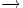$\to$ lower quantity of$B$$\to$ increase in utility of consuming$A$$\to$ increase in quantity of$A$ demanded at a given price
complementary effects$A$ and$B$ are complementary goods: having more of$A$ increases the marginal utility of$B$ and vice versa. A change in the price of$B$ affects the quantity of$B$ demanded. By the law of demand, the quantity demanded decreases with an increase in price. This in turn affects the utility function of$A$, and hence, the quantity demanded at a given price. This tends to make the cross-price elasticity of demand more negative, because increasing price of$B$$\to$ lower quantity of$B$$\to$ decrease in utility of consuming$A$$\to$ decrease in quantity of$A$ demanded at a given price

### Sign

The sign of the cross-price elasticity of demand depends on the relative extent of operation of the various factors that influence the cross-price elasticity of demand noted above. In some cases, the sign is unambiguous, while in others, it is ambiguous.

Is the magnitude of own-price elasticity of demand of$B$ greater than or less than$1$? Do$A$ and$B$ complement or substitute for each other? Conclusion about sign of cross-price elasticity
less than$1$ complement negative
less than$1$ substitute ambiguous but typically positive; positive if substitution effect dominates, which would be the case for close substitutes.
greater than$1$ complement ambiguous; sign depends on whether the complementary effect or income effect is stronger.
greater than$1$ substitute positive